Next: Incompressible Euler equations Up: Central schemes in two Previous: Central schemes in two

## Boundary conditions

Following , we demonstrate our boundary treatment in the case of the left-boundary (see Figure 1.4.10).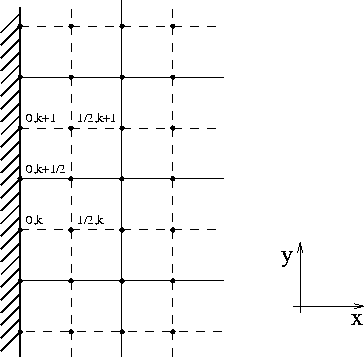Figure 1.4.10: Two dimensions - left boundary

We distinguish between inflow (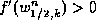), and outflow (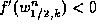), boundary cells.

In inflow boundary cells, we reconstruct a constant interpolant from the prescribed point-values at these boundaries,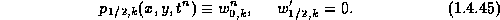This reconstruction is then used to build the approximate solution at time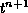in the interior cells. At the next-time step,, the cell-averages at these boundary cells are defined according to the prescribed point-values as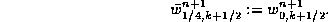We now turn to the outflow boundary cells. Here, we extrapolate the data from the interior of the domain, up to the boundary. First, we determine the discrete slope in the x-direction,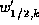. This slope is then used to extrapolate the cell-average up to the boundary,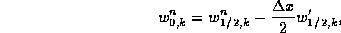which is then used to predict the mid-value,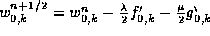. Here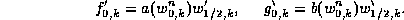The discrete slope in the y-direction,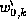, is computed in that boundary cell in an analogous way to the interior computation. In summary, the staggered average at timeis given by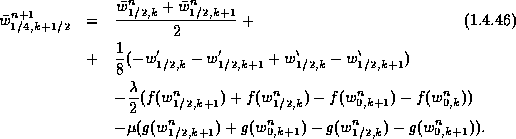This concludes the boundary treatment of the left boundary. Similar expressions hold for the other three boundaries.

We now turn to the corners and as a prototype, consider the upper-left corner (see Figure 1.4.11). In the corner we repeat the previous boundary treatment with one simple modification. The main difference regarding the boundary scheme in the corner is based on the number of different possible inflow/outflow configurations in that corner.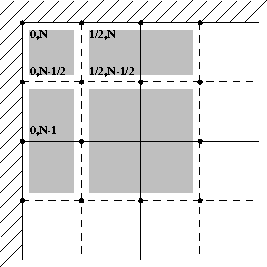Figure 1.4.11: Upper-left corner

Computationally, the most complicated case is when the flow in that upper-left corner is outflow in both directions. In this case, the staggered average at time,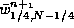, is computed according to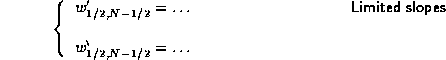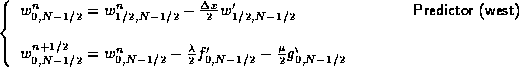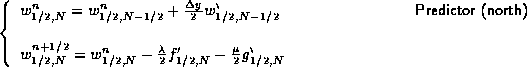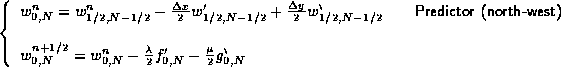The cell-average in the north-west edge of Figure 1.4.11 in time, is given in this outflow-outflow case by the corrector step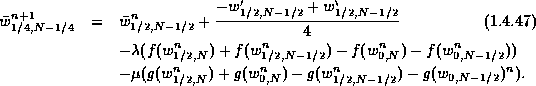When one of the boundaries is inflow, we have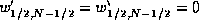, and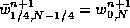(- the prescribed pointvalues at the corner).

As an example, we we approximate a solution to the two-dimensional Burgers equation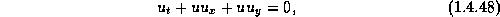subject to the initial conditions,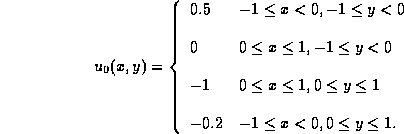and augmented with boundary conditions at the inflow boundaries which are equal to the initial values at these same boundaries. Figures 1.4.12 show the evolution of the solution in time for mesh sizes 41*41 and 81*81. Again, we note that there are no spurious oscillations at the boundaries, oscillations that are inherent with a naive treatment of inflow boundaries.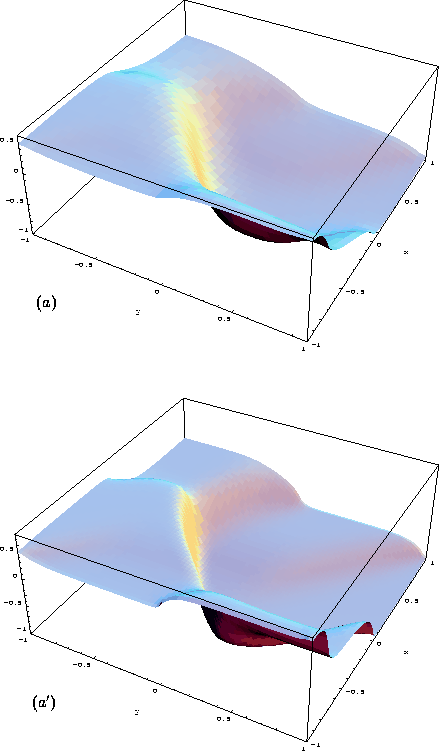Figure 1.4.12: The 2D IBVP Burgers equation: T=1. (a) N=41, (a') N=81Next: Incompressible Euler equations Up: Central schemes in two Previous: Central schemes in two